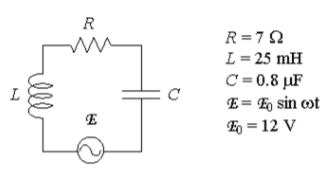# Problem: A series RLC circuit with L = 25 mH, C = 0.8 μF and R = 7Ω is driven by a generator with a maximum emf of 12V and a variable angular frequency ω. At ω = 8000 rad/sec the impedance Z of the circuit is:a) Z = 7 Ωb) Z = 12.3 Ωc) Z = 27.1 Ωd) Z = 44.3 Ωe) Z = 172 Ω

###### FREE Expert Solution
94% (242 ratings)
###### Problem Details

A series RLC circuit with L = 25 mH, C = 0.8 μF and R = 7Ω is driven by a generator with a maximum emf of 12V and a variable angular frequency ω. At ω = 8000 rad/sec the impedance Z of the circuit is:

a) Z = 7 Ω

b) Z = 12.3 Ω

c) Z = 27.1 Ω

d) Z = 44.3 Ω

e) Z = 172 ΩFrequently Asked Questions

What scientific concept do you need to know in order to solve this problem?

Our tutors have indicated that to solve this problem you will need to apply the Impedance in AC Circuits concept. You can view video lessons to learn Impedance in AC Circuits. Or if you need more Impedance in AC Circuits practice, you can also practice Impedance in AC Circuits practice problems.

How long does this problem take to solve?

Our expert Physics tutor, Juan took 1 minute and 48 seconds to solve this problem. You can follow their steps in the video explanation above.